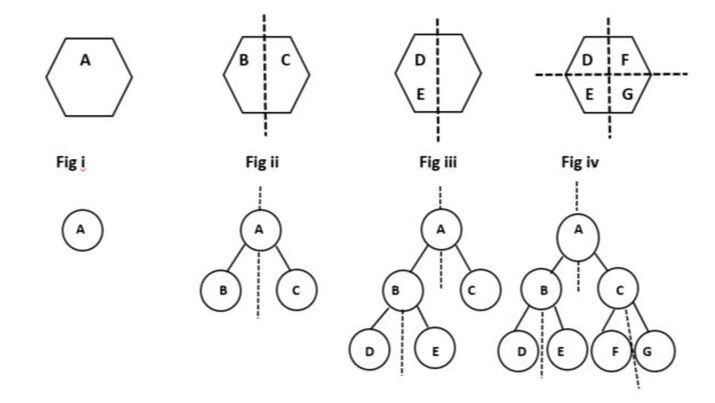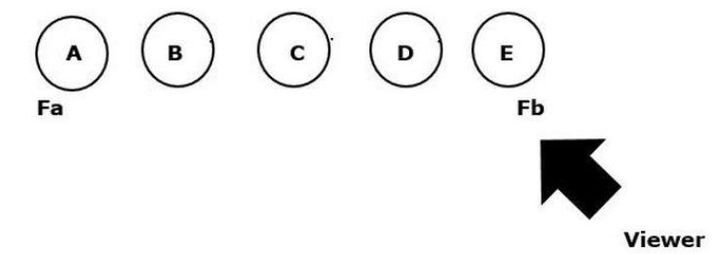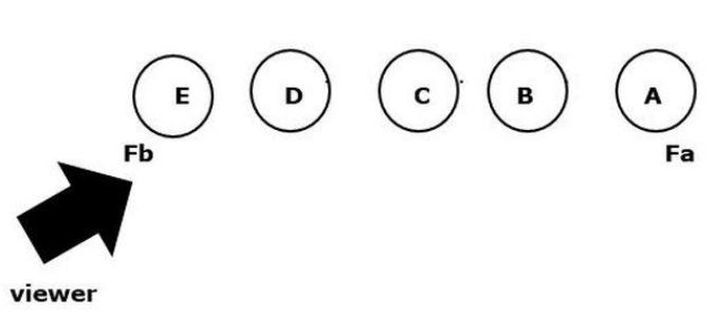# Binary Space Partitioning

A binary space partition is a data structure used in computer graphics and algorithmic geometry to divide a space into smaller parts. BSP was developed in the environment of 3D computer graphics. BSP includes applications such as operation with geometrical shapes, geospatial, and ray tracing.

It is a two-step procedure

Step 1 − Creation of BSP tree.

Step 2 − Display a tree.

## Creation of BSP tree

Visual Representation of Binary Space PartitioningThe binary Space Partitioning algorithm recursively divides the space into two half-spaces. So keep in account that there is a dividing region in every polygon figure.

Understands the steps of creating a BSP in a tree −

• We will apply the algorithm first to the root node of the tree i.e. A.

• We then apply the BSP algorithms B to the left node and C to the right node where A is the parent or root node of its.

• Now we start the leftmost second partitioning with the help of the B node where we simply add the child node D to the left and E to the right node.

• After completing the leftmost node, we start the rightmost second partitioning with the help of the C node where we simply add node F to the left and G to the right node.

## Display a tree

Let’s take an example of a tree traverse,Fa, A, B, C, D, E, Fb --- (LDR)

• If a viewpoint is back, then traverse the tree inorder.

• LDR represents as

Process of left sub-tree -> Process of current-node -> Process of right sub-tree.


Let’s take an example of a tree traverse in reverse order,Fb, E, D, C, B, A, Fa --- (RDL)

• If the viewpoint is front then traverse the tree in reverse or inorder.

• RDL represents as

Process of right sub-tree -> Process of current-node -> Process of left sub-tree.


## Advantages of Binary Space Partitioning

• It is easy to understand and implement the program on a binary tree.

• It can improve scalability and optimize performance.

• Gaming industries take good advantage of BSP by updating or developing the entire game based on BSP.

## Disadvantages of Binary Space Partitioning

• Generating BSP takes lots of time.

• It does not solve the problem of VSD(Visual Surface Detection).

## Conclusion

We all know that Binary Space Partitioning is a data structure, but with its help, we can also create a binary tree, in which all nodes have 2 branches. This technique is useful in making 3D games. For example, the Doom game is very popular and it is being developed using the BSP algorithm.

Updated on: 20-Apr-2023

2K+ Views